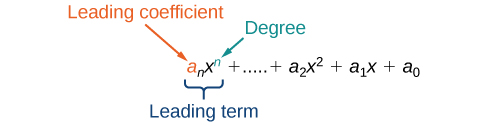# 1.4 Polynomials

 Page 1 / 15
In this section students will:
• Identify the degree and leading coefficient of polynomials.
• Multiply polynomials.
• Use FOIL to multiply binomials.
• Perform operations with polynomials of several variables.

Earl is building a doghouse, whose front is in the shape of a square topped with a triangle. There will be a rectangular door through which the dog can enter and exit the house. Earl wants to find the area of the front of the doghouse so that he can purchase the correct amount of paint. Using the measurements of the front of the house, shown in [link] , we can create an expression that combines several variable terms, allowing us to solve this problem and others like it.

First find the area of the square in square feet.

$\begin{array}{ccc}\hfill A& =& {s}^{2}\hfill \\ & =& {\left(2x\right)}^{2}\hfill \\ & =& 4{x}^{2}\hfill \end{array}$

Then find the area of the triangle in square feet.

Next find the area of the rectangular door in square feet.

$\begin{array}{ccc}\hfill A& =& lw\hfill \\ & =& x\cdot 1\hfill \\ \hfill & =& x\hfill \end{array}$

The area of the front of the doghouse can be found by adding the areas of the square and the triangle, and then subtracting the area of the rectangle. When we do this, we get $\text{\hspace{0.17em}}4{x}^{2}+\frac{3}{2}x-x\text{\hspace{0.17em}}{\text{ft}}^{2},$ or $\text{\hspace{0.17em}}4{x}^{2}+\frac{1}{2}x\text{\hspace{0.17em}}$ ft 2 .

In this section, we will examine expressions such as this one, which combine several variable terms.

## Identifying the degree and leading coefficient of polynomials

The formula just found is an example of a polynomial    , which is a sum of or difference of terms, each consisting of a variable raised to a nonnegative integer power. A number multiplied by a variable raised to an exponent, such as $\text{\hspace{0.17em}}384\pi ,$ is known as a coefficient    . Coefficients can be positive, negative, or zero, and can be whole numbers, decimals, or fractions. Each product $\text{\hspace{0.17em}}{a}_{i}{x}^{i},$ such as $\text{\hspace{0.17em}}384\pi w,$ is a term of a polynomial    . If a term does not contain a variable, it is called a constant .

A polynomial containing only one term, such as $\text{\hspace{0.17em}}5{x}^{4},$ is called a monomial    . A polynomial containing two terms, such as $\text{\hspace{0.17em}}2x-9,$ is called a binomial    . A polynomial containing three terms, such as $\text{\hspace{0.17em}}-3{x}^{2}+8x-7,$ is called a trinomial    .

We can find the degree    of a polynomial by identifying the highest power of the variable that occurs in the polynomial. The term with the highest degree is called the leading term    because it is usually written first. The coefficient of the leading term is called the leading coefficient    . When a polynomial is written so that the powers are descending, we say that it is in standard form.## Polynomials

A polynomial    is an expression that can be written in the form

${a}_{n}{x}^{n}+...+{a}_{2}{x}^{2}+{a}_{1}x+{a}_{0}$

Each real number a i is called a coefficient    . The number $\text{\hspace{0.17em}}{a}_{0}\text{\hspace{0.17em}}$ that is not multiplied by a variable is called a constant . Each product $\text{\hspace{0.17em}}{a}_{i}{x}^{i}\text{\hspace{0.17em}}$ is a term of a polynomial    . The highest power of the variable that occurs in the polynomial is called the degree    of a polynomial. The leading term    is the term with the highest power, and its coefficient is called the leading coefficient    .

Given a polynomial expression, identify the degree and leading coefficient .

1. Find the highest power of x to determine the degree.
2. Identify the term containing the highest power of x to find the leading term.
3. Identify the coefficient of the leading term.

what are odd numbers
numbers that leave a remainder when divided by 2
Thorben
1,3,5,7,... 99,...867
Thorben
7%2=1, 679%2=1, 866245%2=1
Thorben
the third and the seventh terms of a G.P are 81 and 16, find the first and fifth terms.
if a=3, b =4 and c=5 find the six trigonometric value sin
pls how do I factorize x⁴+x³-7x²-x+6=0
in a function the input value is called
how do I test for values on the number line
if a=4 b=4 then a+b=
a+b+2ab
Kin
commulative principle
a+b= 4+4=8
Mimi
If a=4 and b=4 then we add the value of a and b i.e a+b=4+4=8.
Tariq
what are examples of natural number
an equation for the line that goes through the point (-1,12) and has a slope of 2,3
3y=-9x+25
Ishaq
show that the set of natural numberdoes not from agroup with addition or multiplication butit forms aseni group with respect toaaddition as well as multiplication
x^20+x^15+x^10+x^5/x^2+1
evaluate each algebraic expression. 2x+×_2 if ×=5
if the ratio of the root of ax+bx+c =0, show that (m+1)^2 ac =b^2m
By the definition, is such that 0!=1.why?
(1+cosA+IsinA)(1+cosB+isinB)/(cos@+isin@)(cos$+isin$)
hatdog
Mark
jaks
Ryan

#### Get Jobilize Job Search Mobile App in your pocket Now!ByBy OpenStaxBy Jesenia WoffordBy OpenStaxBy Saylor FoundationBy Sarah WarrenBy Rachel CarlisleByBy Brooke DelaneyBy Madison ChristianBy Cath Yu